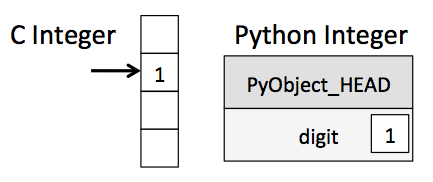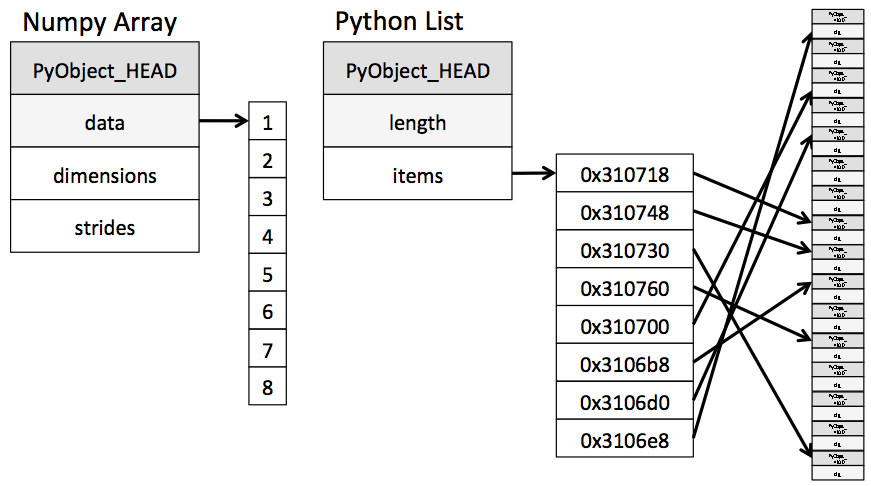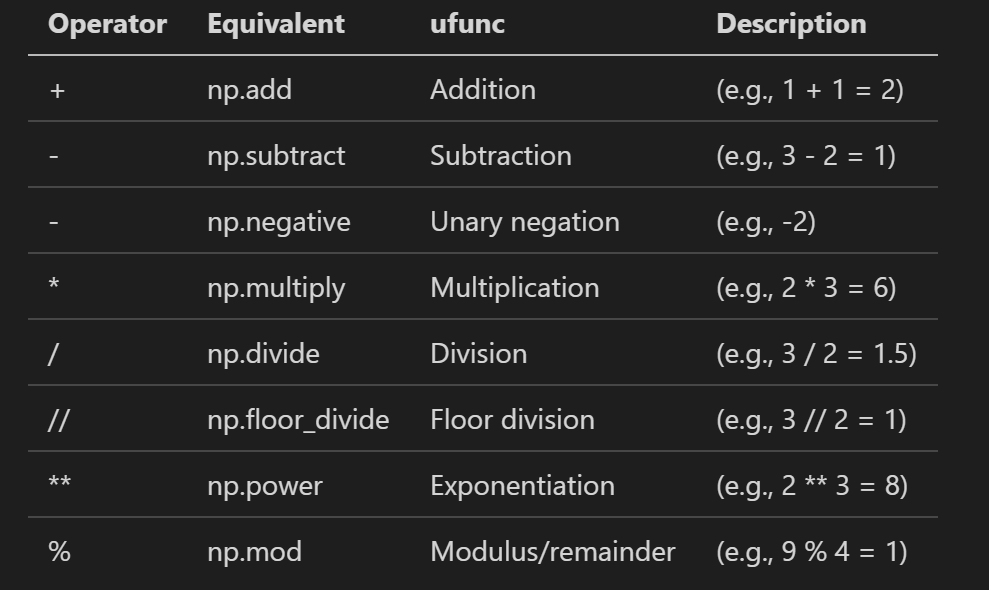# A solution to boost Python speed 1000x times

## People said Python is slow, how slow it can be

Whenever there is a programming speed competition, Python usually goes to the bottom. Some said that is because Python is an interpretation language. All interpretation language is slow. But we know that Java is also a kind of language, its bytecode is interpreted by JVM. As showing in this benchmark, Java is much faster than Python.

Here is a sample that can demo Python's slowness. Use the traditional for-loop to produce reciprocal numbers:

``````import numpy as np
np.random.seed(0)
values = np.random.randint(1, 100, size=1000000)
def get_reciprocal(values):
output = np.empty(len(values))
for i in range(len(values)):
output[i] = 1.0/values[i]
%timeit get_reciprocal(values)
``````

The result:

``````3.37 s ± 582 ms per loop (mean ± std. dev. of 7 runs, 1 loop each)
``````

Holy cow, it takes 3.37s to calculate merely 1,000,000 reciprocal numbers. The same logic in C takes just a blink: 9ms; C# takes 19ms; Nodejs takes 26ms; Java takes 5ms! and Python takes self-doubting 3.37 SECONDS. (I attached all test code in the end).

### The root cause of the slowness

We usually call Python as a dynamic type programming language. And everything in Python program is object, in other words, every time when Python code deals with data, it needs to unbox the object wrapper. Inside of the for loop, each iteration will need to unbox objects, check type and calculate the reciprocal number.** Those 3 seconds are all wasting in the type checking**.

Unlike traditional languages like C, access to data is direct, while in Python, lots of CPU cycles are being used to check the type.Even a simple number assigning will go a long pass.

``````a = 1
``````

Step 1. Set a->PyObject_HEAD->typecode to integer Step 2. Set a->val =1

For more about why Python is slow, it is worthy to read Jake's wonderful article: Why Python is Slow: Looking Under the Hood

So, is there a way to walk around the type checking, hence boost the performance?

### The Solution: NumPy Universal Functions

Unlike Python list, NumPy array is an object build around a C array. Access item in NumPy needs no steps to check types. This brings us light to the solution, it is NumPy Universal Functions aka UFunc.In short, UFunc is a way that we can do arithmetic operations directly to a whole array. Translate the first slow Python sample to UFunc version, it will be like this:

``````import numpy as np
np.random.seed(0)
values = np.random.randint(1, 100, size=1000000)
%timeit result = **1.0/values**
``````

This code not only boosts the speed, and also make the code shorter. Guess how much time it takes now? 2.7ms faster than any other languages I mentioned above:

``````2.71 ms ± 50.8 µs per loop (mean ± std. dev. of 7 runs, 100 loops each)
``````

Go back to the code, the key is the 1.0/values. values here is not a number, it is a NumPy array. Like the divide operator, there are many others.Check here for all Ufunc operators.

### Summary

For those using Python, high chances you are using Python dealing with data and numbers. Those data can be stored in NumPy or Pandas DataFrame, since DataFrame is implemented based on NumPy. So, Ufunc works too.

The UFunc enable us perform repeating operations in Python faster in orders of magnitude. The slowest Python can even faster than C language. That is cool.

### Appendix — test code for C, C#, Java, and NodeJS

C Language:

``````#include <stdio.h>
#include <stdlib.h>
#include <sys/time.h>

int main(){
struct timeval stop, start;
int length = 1000000;
int rand_array[length];
float output_array[length];
for(int i = 0; i<length; i++){
rand_array[i] = rand();
}
gettimeofday(&start, NULL);
for(int i = 0; i<length; i++){
output_array[i] = 1.0/(rand_array[i]*1.0);
}
gettimeofday(&stop, NULL);
printf("took %lu us\n", (stop.tv_sec - start.tv_sec) * 1000000 + stop.tv_usec - start.tv_usec);
printf("done\n");
return 0;
}
``````

C#(dotnet 5.0):

``````using System;
namespace speed_test{
class Program{
static void Main(string[] args){
int length = 1000000;
double[] rand_array =new double[length];
double[] output = new double[length];
var rand = new Random();
for(int i =0; i<length;i++){
rand_array[i] = rand.Next();
//Console.WriteLine(rand_array[i]);
}
long start = DateTimeOffset.Now.ToUnixTimeMilliseconds();
for(int i =0;i<length;i++){
output[i] = 1.0/rand_array[i];
}
long end = DateTimeOffset.Now.ToUnixTimeMilliseconds();
Console.WriteLine(end - start);
}
}
}
``````

Java:

``````import java.util.Random;

public class speed_test {
public static void main(String[] args){
int length = 1000000;
long[] rand_array = new long[length];
double[] output = new double[length];
Random rand = new Random ();
for(int i =0; i<length; i++){
rand_array[i] = rand.nextLong();
}
long start = System.currentTimeMillis();
for(int i = 0;i<length; i++){
output[i] = 1.0/rand_array[i];
}
long end = System.currentTimeMillis();
System.out.println(end - start);
}
}
``````

NodeJS:

``````let length = 1000000;
let rand_array = [];
let output = [];
for(var i=0;i<length;i++){
rand_array[i] = Math.floor(Math.random()*10000000);
}
let start = (new Date()).getMilliseconds();
for(var i=0;i<length;i++){
output[i] = 1.0/rand_array[i];
}
let end = (new Date()).getMilliseconds();
console.log(end - start);
``````